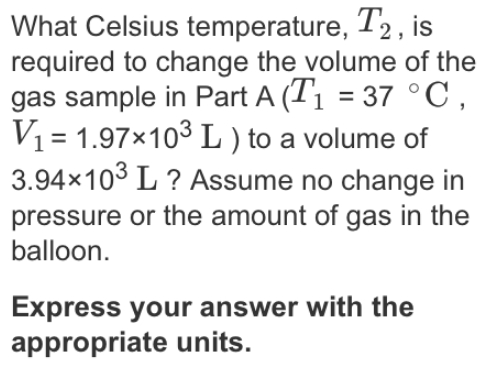# Problem: What Celsius temperature, T2, is required to change the volume of the gas sample in Part A (T1 = 37°C, V1 = 1.97 x 103 L) to a volume of 3.94 x 103 L? Assume no change in pressure or the amount of gas in the balloon. Express your answer with the appropriate units.

###### FREE Expert Solution
83% (50 ratings)###### Problem Details

What Celsius temperature, T2, is required to change the volume of the gas sample in Part A (T1 = 37°C, V1 = 1.97 x 103 L) to a volume of 3.94 x 103 L? Assume no change in pressure or the amount of gas in the balloon.